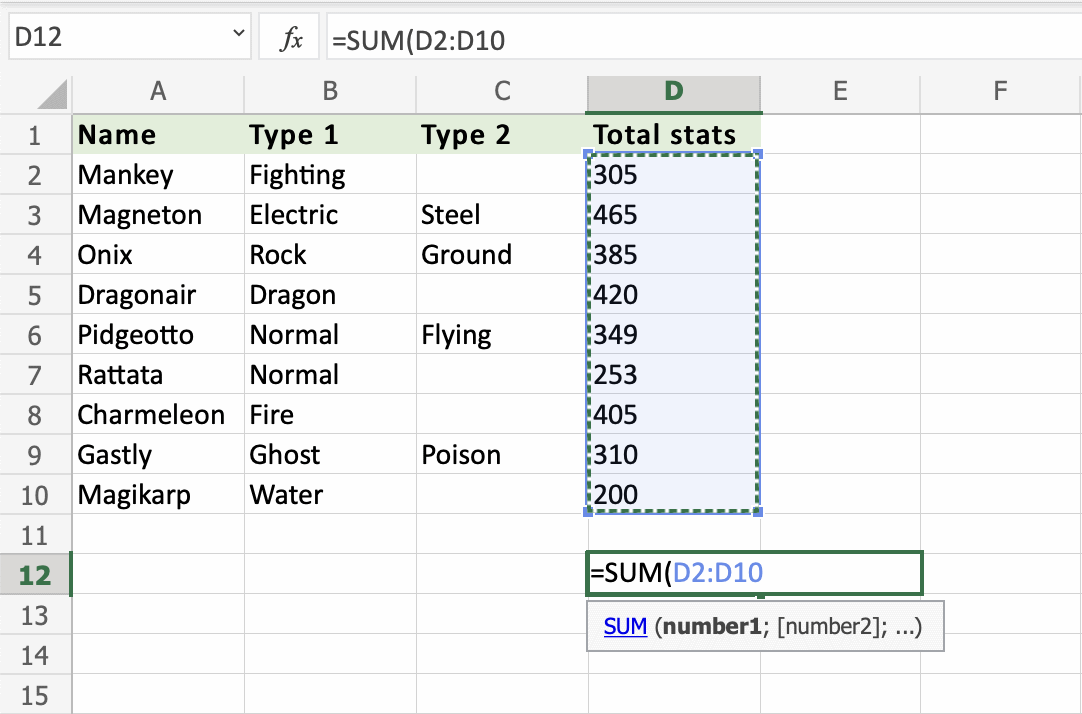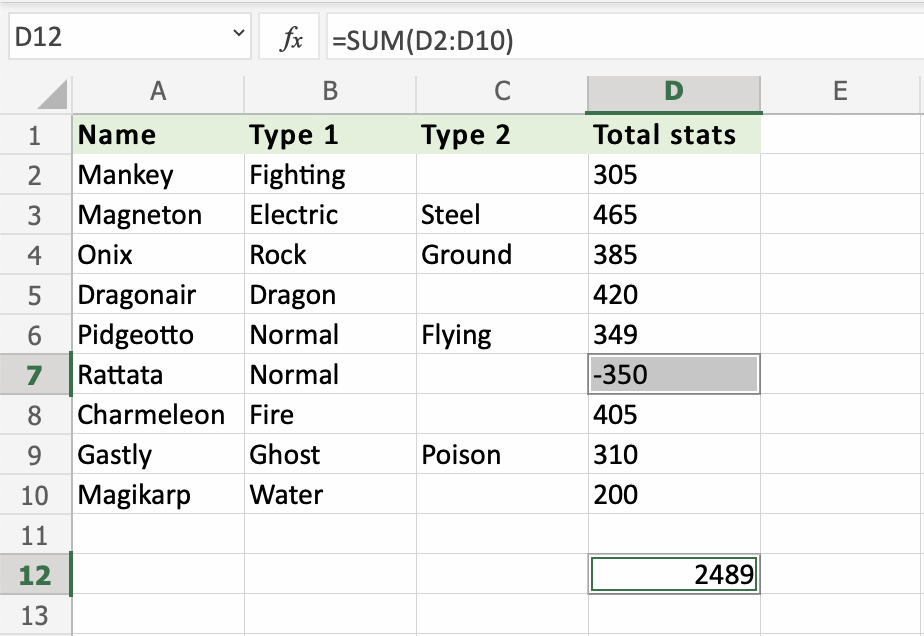# Excel SUM Function

## SUM Function

The SUM function is a premade function in Excel, which adds numbers in a range.

It is typed `=SUM`

Note: The `=SUM` function adds cells in a range, both negative and positive.

How to use the `=SUM` function:

1. Select a cell
2. Type `=SUM`
3. Double click the SUM command
4. Select a range
5. Hit enter

Let's see some examples!Copy ValuesGreat job! You have successfully calculated the sum of the stats using the `=SUM` function. The Pokemon's stats have a total sum of `3092`.

Let's change one of the values in the range, to see what happens. Type `D7(350)`:Rattata total stats was changed from `(D7)253` to `(D7)350`.

The SUM function updated the total sum from `D12(3092)` to `D12(3189)`.

It updates the SUM when values inside of the range are changed.

Lets change Rattata stats to a negative number to see what happens. Type `D7(-350)`:The SUM is updated to `D12(2489)`. Adding a negative number subtracts that value from the range.

The `=SUM` function adds both positive and negative numbers.

W3Schools is optimized for learning and training. Examples might be simplified to improve reading and learning. Tutorials, references, and examples are constantly reviewed to avoid errors, but we cannot warrant full correctness of all content. While using W3Schools, you agree to have read and accepted our terms of use, cookie and privacy policy.